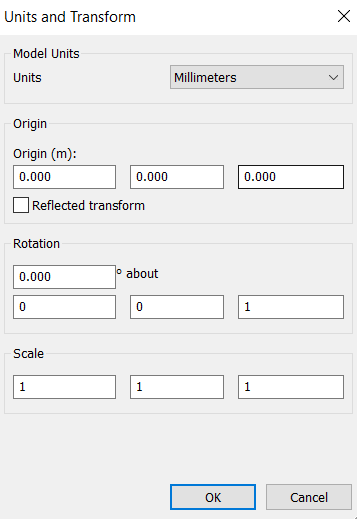# Setting "Units and Transform" values via Navisworks API

Question: I'm trying to modify the Model Units, Origin, Rotation, and scale values of each appended 3D model. Is it possible to change these values via Navisworks API?Answer: Yes, it is possible to change these values using SetModelUnitsAndTransform Method. Please take a look at the below sample code

```
DocumentModels models = doc.Models;
//Get the required model from DocumentModels
Model model;

Transform3D oldTransform3d=model.Transform;
Transform3DComponents transform3dComponents = oldTransform3d.Factor();

//Get Values
Vector3D originVector3D = transform3dComponents.Translation;
Vector3D scaleVector3D = transform3dComponents.Scale;
Rotation3D rotationVector3D = transform3dComponents.Rotation;

//Set Values
transform3dComponents.Translation = new Vector3D(origin_X, origin_Y, origin_Z);  //Eg: new Vector3D(10,10,10);
transform3dComponents.Rotation = new Rotation3D(new UnitVector3D(0, 0, 1),...```